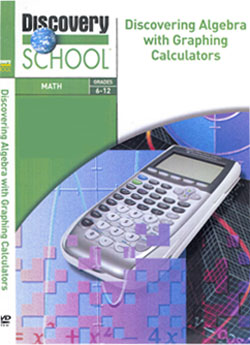## Discovery EducationDiscovery Education has created a premiere video series for use in the algebra classroom. Discovering Math with Graphing Calculators is a tutorial on the use of graphing calculators to learn and apply math, as well as a dynamic documentary series that explains how math is used to solve real-world problems.

Written and hosted by renowned mathematics instructor Monica Neagoy, Discovering Algebra with Graphing Calculators leads students through graphing calculator functions for solving a multitude of algebraic problems. Colorful graphics and animation enliven the presentations, and individuals in a wide range of careers demonstrate the crucial role of math in their everyday lives

The TI-84 Plus Silver Edition series is used throughout the 10 part series.

Graphing a Line
This program shows how to graph a line on the graphing calculator. Students learn step-by-step keystrokes, geometric and algebraic definitions of a linear function, and new visual ways to solve linear equations. A mountain climber explains how math factors into planning a climb.

Finding the Slope of a Line
The video shows how slope measures change in linear relationships. Students learn use of the graphing calculator to find the slope using graphs, a selection of two points, or an equation. A pilot discusses the importance of slope in flying a plan.

This program details quadratic regression and properties for determining heights and roots. Students learn practical applications of the irrational number phi. A roller coaster designer reveals how quadratic function graphs, or parabolas, figure into the engineering of these thrill rides.

Solving Systems of Equations
This program illustrates functions for solving systems of linear and quadratic equations. Using matrices students solve equations in a time-efficient manner. A chef shows how mathematics keeps things cooking at his restaurant.

Investigating Inequalities
This video investigates linear and nonlinear inequalities with one and two variables. Students factor quantity and resource constraints to resolve linear programming problems. A toy manufacturer relates the process of balancing product quality with maximizing profit.

Understanding Exponential Functions
This video investigates the properties of exponential functions and graphs. Students learn the algebra of exponential functions including growth and decay. A banker supplies "interesting" information on how money accrues in bank accounts.

Investigating Logarithmic Functions
This video investigates logarithmic functions and their use in astronomy and other sciences. Students learn to graph logarithmic functions and their inverses. An archaeologist reveals how logarithms factor into radiocarbon dating of artifacts.

Plotting the Curve of Best Fit
This program examines plotting data to determine a curve (linear, quadratic, or exponential) of best fit. Students create scatter plots, interpret results, and make predictions based on table values. A university swim coach and team members relate how math factors into their training and results.

Examining Probability
This program demonstrates solving problems of probability. Students learn the use of the graphing calculator's random number generator to verify predictions. Probability factors significantly into weather forecasting and a meteorologist shows how math helps predict sunshine or showers. (To access the Chaos game referenced in this video - please click here -    CHAOS)

Representing and Analyzing Data
This program demonstrates representing and analyzing data, making inferences, and drawing conclusions. Students learn to create line graphs, histograms, and other mathematical forms for data analysis. A volcanologist relates how data analysis helps determine the nature of volcanic eruptions.